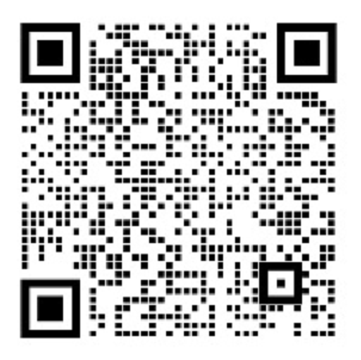News Information

<section powered-by="xiumi.us" style="margin: 0px; padding: 0px; outline: 0px; max-width: 100%; font-family: system-ui, -apple-system, BlinkMacSystemFont, " helvetica="" neue",="" "pingfang="" sc",="" "hiragino="" sans="" gb",="" "microsoft="" yahei="" ui",="" yahei",="" arial,="" sans-serif;="" letter-spacing:="" 0.5px;="" text-align:="" justify;="" color:="" rgb(79,="" 79,="" 79);="" font-size:="" 15px;="" box-sizing:="" border-box="" !important;="" overflow-wrap:="" break-word="" !important;"="">